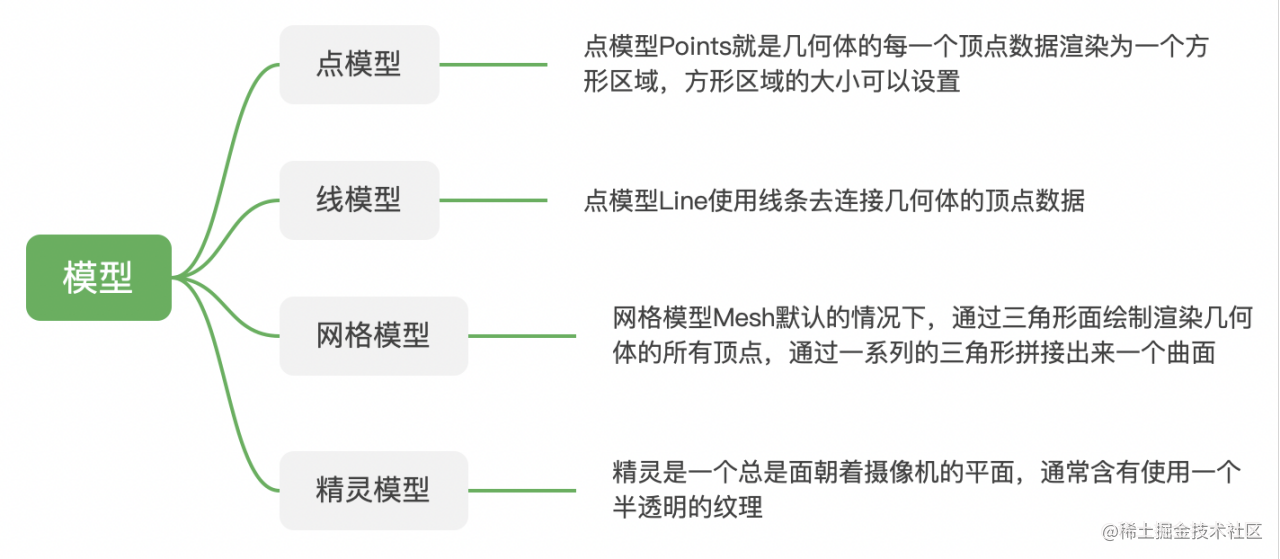# 1. three.js基础回顾

## 1.1 three.js概览

1. 是什么？
Three.js 是一款 webGL 框架，它封装了底层的图形接口，使得能够在无需掌握繁冗的图形学知识的情况下，也能用简单的代码实现三维场景的渲染。

2. 核心

• 渲染器: 将场景中的物体进行渲染
• 场景: 场景是所有物体的容器，也对应着我们创建的三维世界
• 相机: 一种投影方式，将三维的场景显示到二维的屏幕上

## 1.2 相机

1. 是什么

2. 分类

• 正交投影照相机: 对于在三维空间内平行的线，投影到二维空间中也一定是平行的
• 透视投影照相机: 有近大远小的效果

## 1.3 模型

1. 主要有以下几种模型2. 模型包括什么

• 几何形状
• 材质

1. 分类

• 环境光

• 点光源

• 平行光

• 聚光灯

• 阴影

# 2. 全景图的实现

• 建立球体模型并令照相机位于球体中
• 不断移动照相机的位置使其观察到球体内的各个角度

## 2.1 建立基本场景

• 初始化渲染器
``````const setupRenderer = () => {
const renderer = new THREE.WebGLRenderer({antialias: true})
renderer.setSize(window.innerWidth, window.innerHeight)
document.body.appendChild(renderer.domElement)
return renderer
}

• 初始化相机
``````// 建立透视投影相机制造出“近大远小”的效果
const setupCamera = () => {
const aspectRatio = window.innerWidth / window.innerHeight
const camera = new THREE.PerspectiveCamera(90, aspectRatio, 0.0001, 10000)
camera.position.set(window.obj.camerax, window.obj.cameray, window.obj.cameraz)

return camera
}

• 初始化场景scene和辅助线
``````  const scene = new THREE.Scene()
const axesHelper = new THREE.AxesHelper(1000)
const cameraHelper = new THREE.CameraHelper(camera)
const gridHelper = new THREE.GridHelper(1000, 10)

// 布置场景

• 建立球体模型
``````// 采用加载纹理贴图的方式将一张普通的全景图贴在球体的表面
const sphereMaterial = new THREE.MeshBasicMaterial({
map: texture,
side: THREE.DoubleSide,
})
const sphere = new THREE.Mesh(sphereGeo, sphereMaterial)

## 2.2 核心：鼠标移动-相机移动

1. 涉及到以下几点：

• 监听鼠标的按下、移动事件
• 鼠标移动距离转化成经纬度
• 经纬度转化成相机的坐标

2. 代码解读

• 监听鼠标事件
``````// mousedown事件，记录移动的起点
startPosX = e.clientX
startPosY = e.clientY
startTs = Date.now()
isMouseDown = true
})
// mousemove, 记录移动的终点，并调用move函数进行实时计算
if (!isMouseDown) return
const posX = e.clientX
const posY = e.clientY
const curTs = Date.now()

// 根据起始和移动过程中的坐标、移动的时间来移动相机
let startPos = { x: startPosX, y: startPosY };
let endPos = { x: posX, y: posY };
let duration = curTs - startTs;

move(startPos, endPos, duration)

// 以当前的终点为下一次的起点
startPosX = posX
startPosY = posY
startTs = curTs
})

• move函数的实现：根据转化出来的相机坐标实时的设置相机的位置
• 鼠标移动距离->经纬度
• 经纬度-坐标
``````const move = (startPos, endPos, duration) => {
if (duration === 0) return

const { x: sx, y: sy } = startPos
const { x: ex, y: ey } = endPos
const vx = (ex - sx) / duration// X 轴方向上的速度
const vy = (ey - sy) / duration// Y 轴方向上的速度
// 1.鼠标移动距离->经纬度
const { longtitude, latitude } = getMovPos({
startPos,
endPos: { x: vx * moveBuffTime + ex, y: vy * moveBuffTime + ey },
curRotate: { longtitude: movObj.longtitude , latitude: movObj.latitude },
})

// 设置移动参数
// 在update中设置相机实时的坐标会使移动过程更细腻
const gsapOpts = {
ease: "power4.out",
duration: 1,
onUpdate: () => {
// 2.经纬度->相机的坐标
const { latitude: newLati, posObj: newPos } = setCameraPos({
longtitude: movObj.longtitude,
latitude: movObj.latitude
});

movObj.latitude = newLati;
movObj.longtitude = longtitude;

// 3. 设置摄像机坐标
posObj.camerax = newPos.x;
posObj.cameray = newPos.y;
posObj.cameraz = newPos.z;
},

};
gsap.to(movObj, gsapOpts);
}

``````const { latitude: newLatitude, posObj: newPos } = setCameraPos({longtitude: movObj.longtitude, latitude: movObj.latitude})
// 3.移动相机
gsap.to(posObj, {
ease: 'power4.out',
duration: 1,
camerax: newPos.x,
cameray: newPos.y,
cameraz: newPos.z,
})
movObj.longtitude = longtitude
movObj.latitude = latitude

• 鼠标移动距离->经纬度

``````const { x: sx, y: sy } = startPos
const { x: ex, y: ey } = endPos
const { longtitude: curLongtitude, latitude: curLatitude } = curRotate

const longtitude = (sx - ex) * 0.138 + curLongtitude
const latitude = (sy - ey) * 0.12 + curLatitude
return {
longtitude,
latitude,
}

• 经纬度->坐标
• 角度->弧度：三角函数计算用到的是弧度
• 涉及到了数学上的计算：已知球体的半径，计算空间中的某一点的坐标
``````function setCameraPos ({latitude,longtitude}) {
const newLatitude = Math.max(-85, Math.min(85, latitude))
//将经纬度转化为弧度
const posObj = {
x: 0,
y: 0,
z: 0,
}
// 关键公式计算
const r = 100;
posObj.y = r * Math.sin(phi);
const ob = r * Math.cos(phi);
posObj.z = ob * Math.cos(theta);
posObj.x = ob * Math.sin(theta);

return {
latitude: newLatitude,
posObj,
}
}

OB = OD * cos(∠DOB)
D在x轴上的距离就是OC=AB=OB * sin(∠AOB)
D在Z轴上的距离就是Oa=OB *cos(∠AOB)